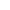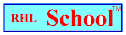Name_______________________________________________Date_________________________
Mathematics Problem Solving
Volume 6, Number 15, January 22, 2001
www.rhlschool.com

# Mean and Median

The mean of a set of numbers is the average. You find the average by adding all the numbers and dividing the sum by the amount of numbers you added.

The median of a set of numbers is the middle number. If you list all the numbers in order, the median is in the middle of the list. If it’s an even amount of numbers, average the two numbers in the middle.

Examples:

These are Ryan’s spelling test scores:

38, 75, 20, 90, 77

To find the mean (average) score, first you add the scores.

38 + 75 + 20 + 90 + 77 = 300

There are 5 scores, so divide 300 by 5. 300 divided by 5 = 60.

Ryan’s mean score is 60.

To find the median score, put the scores in order.

20,38, 75, 77, 90or90, 77, 75, 38, 20

The middle score is 75, so Ryan’s median score is 75.rhlschool.comRHL School - Free Worksheets and MoreCopyright 2001 RHLName_______________________________________________Date_________________________
Mathematics Problem Solving
Volume 6, Number 15, January 22, 2001
www.rhlschool.com

# Mean and Median

Find the mean and median of the following sets of numbers.

1.8, 400, 12

The mean is ________. The median is ________.

2.10, 6, 8

The mean is ________. The median is ________.

3.5, 31, 88, 31, 100

The mean is ________. The median is ________.

4.10, 20,30, 40, 50, 60

The mean is ________. The median is ________.rhlschool.comRHL School - Free Worksheets and MoreCopyright 2001 RHL

 Tweet GDelicious E-mail

Math Problem Solving Home

RHL School Home